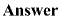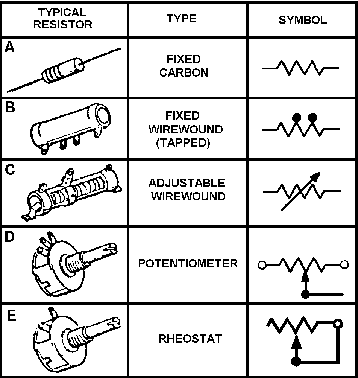magnitude of resistance are exactly the same for conductance, but they affect conductance in the opposite manner."> Conductance - Page 1pCustom SearchMeasurement of CurrentMatter, Energy, and ElectricityWattage RatingCONDUCTANCE

Electricity is a study that is frequently explained in terms of opposites. The term that is the opposite of resistance is CONDUCTANCE. Conductance is the ability of a material to pass electrons. The factors that affect the magnitude of resistance are exactly the same for conductance, but they affect conductance in the opposite manner. Therefore, conductance is directly proportional to area, and inversely proportional to the length of the material. The temperature of the material is definitely a factor, but assuming a constant temperature, the conductance of a material can be calculated.
The unit of conductance is the MHO (G), which is ohm spelled backwards. Recently the term mho has been redesignated SIEMENS (S). Whereas the symbol used to represent resistance (R) is the Greek letter omega (W), the symbol used to represent conductance (G) is (S). The relationship that exists between resistance (R) and conductance (G) or (S) is a reciprocal one. A reciprocal of a number is one divided by that number. In terms of resistance and conductance:

 R = 1 , G = 1 — — G R

Q54.What is the unit of conductance and what other term is sometimes used?Q55.What is the relationship between conductance and resistance?ELECTRICAL RESISTORS

Resistance is a property of every electrical component. At times, its effects will be undesirable. However, resistance is used in many varied ways. RESISTORS are components manufactured to possess specific values of resistance. They are manufactured in many types and sizes. When drawn using its schematic representation, a resistor is shown as a series of jagged lines, as illustrated in figure 1-29.Figure 1-29. - Types of resistors.

Q56.What is schematic symbol for a resistor?Composition of Resistors

One of the most common types of resistors is the molded composition, usually referred to as the carbon resistor. These resistors are manufactured in a variety of sizes and shapes. The chemical composition of the resistor determines its ohmic value and is accurately controlled by the manufacturer in the development process. They are made in ohmic values that range from one ohm to millions of ohms. The physical size of the resistor is related to its wattage rating, which is the ability of resistor to dissipate heat caused by the resistance.

Carbon resistors, as you might suspect, have as their principal ingredient the element carbon. In the manufacturer of carbon resistors, fillers or binders are added to the carbon to obtain various resistor values. Examples of these fillers are clay, bakelite, rubber, and talc. These fillers are doping agents and cause the overall conduction characteristics to change.
Carbon resistors are the most common resistors found because they are easy to manufacturer, inexpensive, and have a tolerance that is adequate for most electrical and electronic applications. Their prime disadvantage is that they have a tendency to change value as they age. One other disadvantage of carbon resistors is their limited power handling capacity.
The disadvantage of carbon resistors can be overcome by the use of WIREWOUND resistors (fig. 1-29 (B) and (C)). Wirewound resistors have very accurate values and possess a higher current handling capability than carbon resistors. The material that is frequently used to manufacture wirewound resistors is German silver which is composed of copper, nickel, and zinc. The qualities and quantities of these elements present in the wire determine the resistivity of the wire. (The resistivity of the wire is the measure or ability of the wire to resist current. Usually the percent of nickel in the wire determines the resistivity.) One disadvantage of the wirewound resistor is that it takes a large amount of wire to manufacture a resistor of high ohmic value, thereby increasing the cost. A variation of the wirewound resistor provides an exposed surface to the resistance wire on one side. An adjustable tap is attached to this side. Such resistors, sometimes with two or more adjustable taps, are used as voltage dividers in power supplies and other applications where a specific voltage is desired to be "tapped" off.

Q57.What does the wattage rating of a resistor indicate?Q58.What are the two disadvantages of carbon-type resistors?Q59.What type resistor should be used to overcome the disadvantages of the carbon resistor?Fixed and Variable Resistors

There are two kinds of resistors, FIXED and VARIABLE. The fixed resistor will have one value and will never change (other than through temperature, age, etc.). The resistors shown in A and B of figure 1-29are classed as fixed resistors. The tapped resistor illustrated in B has several fixed taps and makes more than one resistance value available. The sliding contact resistor shown in C has an adjustable collar that can be moved to tap off any resistance within the ohmic value range of the resistor.

There are two types of variable resistors, one called a POTENTIOMETER and the other a RHEOSTAT (see views D and E of fig. 1-29.)An example of the potentiometer is the volume control on your radio, and an example of the rheostat is the dimmer control for the dash lights in an automobile. There is a slight difference between them. Rheostats usually have two connections, one fixed and the other moveable. Any variable resistor can properly be called a rheostat. The potentiometer always has three connections, two fixed and one moveable. Generally, the rheostat has a limited range of values and a high current-handling capability. The potentiometer has a wide range of values, but it usually has a limited current-handling capability. Potentiometers are always connected as voltage dividers. (Voltage dividers are discussed in Chapter 3.)

Q60.Describe the differences between the rheostat connections and those of the potentiometer.Q61.Which type of variable resistor should you select for controlling a large amount of current?Privacy Statement - Copyright Information. - Contact Us